# How to make choropleth maps with R

There are many tools to make choropleths out there, each offering various levels of difficulty, and with various advantages. There are cartodb and mapbox which are great for creating server-“baked” tilesets, leaflet and d3.js for making client-side visualizations with html, css, and javascript. My favorite, particularly at the prototyping stage, however, continues to be R and ggplot2. The awesome thing with R choropleths are that, with some practice:

• They are the fastest to prototype and iterate on.
• You are making maps within the R environment, so prototyping not just the look of the map, but also what data feeds into the map, become super easy.

With that introducation, let us begin. (Might as well install the full list of packages used in this example at this point–type install.packages(c("plyr", "ggplot2","rgeos", "maptools"))) in your R window).

## The data we will be mapping today

I started this exercise with a dataset from Nepal's Office of the Controller of Examinations, which shows pass / fail percentages and some other data for the SLC “School-Leaving Certificate”) in Nepal, a national examination that takes place at the end of 10th grade. The data is for the Nepali years 2062 and 2063 (2062 is mid-April 2005 to April 2006, 2063 is 2006-7), because those are the only digital versions accessible to us.

Acrobat was able to scan these pdfs into Excel files for us, which we lightly cleaned up to produce the dataset that you can also find in this repository.

# READ IN DATA

# WHAT COLUMNS DO WE HAVE?
library(plyr)
colwise(class)(edu63)

##   School.Number School.Code   Name District     ENG     NEP    MATH
## 1       integer     integer factor   factor numeric numeric numeric
##       SCI     SOC     HPE     TAP     SAP    DIST   FIRST  SECOND   THIRD
## 1 numeric numeric numeric integer integer integer integer integer integer
##      PASS    FAIL PASS.PERCENT     SEM
## 1 integer integer      numeric numeric

# more detailed output of 'structure' can be gotten with str(edu62), I'm
# using the above code to have a more concise output


So, basically, what we have are data, by school (with school code and name), as well as by district, the total number of students, and number of folks passing various subjects, as well as the column we'll look at deeply here PASS.PERCENT, or percentage of students attending the exams who passed the SLC.

Okay, now to get some maps. GADM.org is a great resource for administrative boundaries of countries around the world–I will go ahead and download the “Admin Boundary Level 3” shapefiles for Nepal from GADM, which correspond to districts we have in the dataset.

Next, we load the map into R. To do this, we'll use the maptools packages, which also needs rgeos installed. You might want to install these by doing install.packages(c("rgeos", "gpclib", "maptools", "sp")) (I've included the sp package while we're at it–this is a package that provides map represnetations shared by many other packages.)

library(rgeos)
library(maptools)
library(gpclib)  # may be needed, may not be

# MAP
plot(np_dist)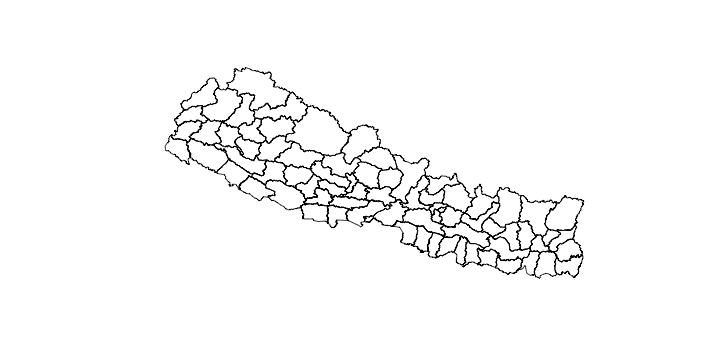## Choropleth iteration I

We'll now generate our first choropleth–which will need plenty of revision–just to demonstrate the method in ggplot2! The method is to first fortify the map object we have read in, where we provide to ggplot the name of a region (for us, this is district, ie, NAME_3 for admin level 3). We'll also go ahead and uppercase the name of the districts because that is how our data names districts, and just try and produce something.

library(ggplot2)
np_dist <- fortify(np_dist, region = "NAME_3")
np_dist$id <- toupper(np_dist$id)  #change ids to uppercase
ggplot() + geom_map(data = edu63, aes(map_id = District, fill = PASS.PERCENT),
map = np_dist) + expand_limits(x = np_dist$long, y = np_dist$lat)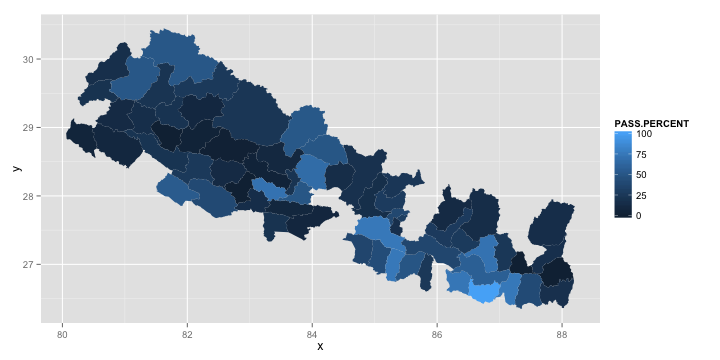If you look at that command in detail, you'll notice that there isn't much there; we are passing in the data, saying that the “District” column should be mapped to the map's ids (which are districts), specifying the map source, and making sure that the boundaries are correct.

Of course, the map looks horrible right now. There are three major issues with it:

1. there is missing data!
2. its not that pretty right now
3. i'm not sure what data we are plotting

So lets deal with each in reverse order.

## Mapping the right data

Our dataset is not a dataset with a row per district, it is a dataset with a row per school. To make sure that we are mapping the right data, we should summarize our data by district, and then map this aggregated data. I like to use ddply for this kind of stuff, which you should read about here if you'd like.

# Take the mean of PASS.PERCENT by District
districtpassavg63 <- ddply(edu63, .(District), summarize, PassMean63 = mean(PASS.PERCENT))
# Same plot, but use the right dataset and fill parameter
ggplot() + geom_map(data = districtpassavg63, aes(map_id = District, fill = PassMean63),
map = np_dist) + expand_limits(x = np_dist$long, y = np_dist$lat)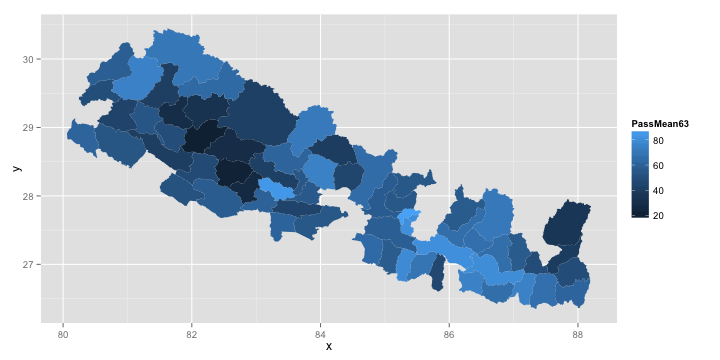## Making it prettier

ggplot allows pretty fancy colorations to be applied to the map. For this example, I think a divergent color scheme works perfectly. After some reading of ggplot docs, the solution for this is quite easy.

ggplot() + geom_map(data = districtpassavg63, aes(map_id = District, fill = PassMean63),
map = np_dist) + expand_limits(x = np_dist$long, y = np_dist$lat) + scale_fill_gradient2(low = muted("red"),
mid = "white", midpoint = 50, high = muted("blue"), limits = c(0, 100))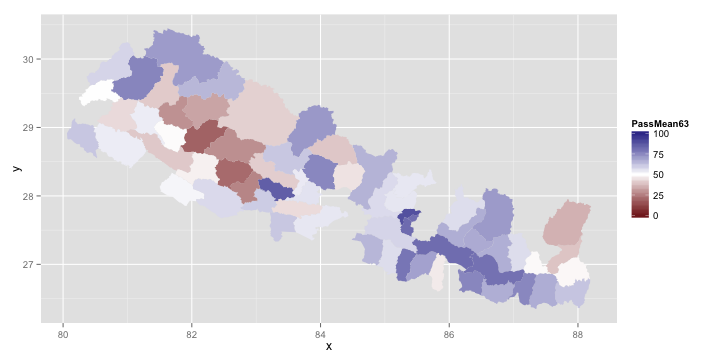## Dealing with missing data

And finally, we come to the most annoying problem, that of missing data. This is a pretty common problem, and comes from the fact that we are matching our dataset to the gadm map using the district name. The two datasets are using different spellings, and this is causing some of the data to be mismatched. Dealing with this kind of mismatch is a pain, and the best solution is usually dependent on the datasets themselves. Here, let us just start by inspecting what is not matching.

namesInData <- levels(edu63$District) namesInMap <- levels(factor(np_dist$id))

namesInData[which(!namesInData %in% namesInMap)]

##   "BARDIA"       "CHITWAN"      "DANDELDHURA"  "DHANUSHA"
##   "KAPILVASTU"   "KAVREPALANCH" "SANKHUWASAB"  "SINDHUPALCHO"
##   "TANAHUN"      "TEHRATHUM"

namesInMap[which(!namesInMap %in% namesInData)]

##   "BARDIYA"        "CHITAWAN"       "DADELDHURA"     "DHANUSA"
##   "KAPILBASTU"     "KAVREPALANCHOK" "SANKHUWASABHA"  "SINDHUPALCHOK"
##   "TANAHU"         "TERHATHUM"


The spelling differences are pretty minor, and thankfully, towards the ends of words. In this case, that means that we can take advantage of alphabetization to solve the problem easily; we'll just re-assign the “levels” of the districts in the data to those of the map, and try the graph now. Note that this may or may not work for your dataset, you may need to resort to anything ranging from find-and-replace in your dataset to name things according to the map, renaming your map admin levels by hand to match your data, or putting in a third “id” parameter that matches between map and data–this is where your data creativeness needs to step in :)

levels(districtpassavg63$District) <- levels(factor(np_dist$id))
ggplot() + geom_map(data = districtpassavg63, aes(map_id = District, fill = PassMean63),
map = np_dist) + expand_limits(x = np_dist$long, y = np_dist$lat) + scale_fill_gradient2(low = muted("red"),
mid = "white", midpoint = 50, high = muted("blue"), limits = c(0, 100))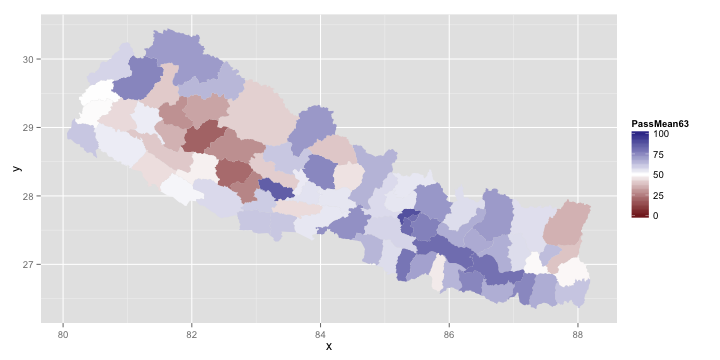## The power of doing this in R – What about 2062? Or the difference?

Of course, the power of doing this in R is that this work is easily repeatable, and we can apply what we did above with just a couple of lines to a new dataset, 2063 data.

edu62 <- read.csv("~/Downloads/fstat-062 reformatted.csv")
levels(edu62$District) <- levels(factor(np_dist$id))
districtpassavg62 <- ddply(edu62, .(District), summarize, PassMean62 = mean(PASS.PERCENT))
ggplot() + geom_map(data = districtpassavg62, aes(map_id = District, fill = PassMean62),
map = np_dist) + expand_limits(x = np_dist$long, y = np_dist$lat) + scale_fill_gradient2(low = muted("red"),
mid = "white", midpoint = 50, high = muted("blue"), limits = c(0, 100))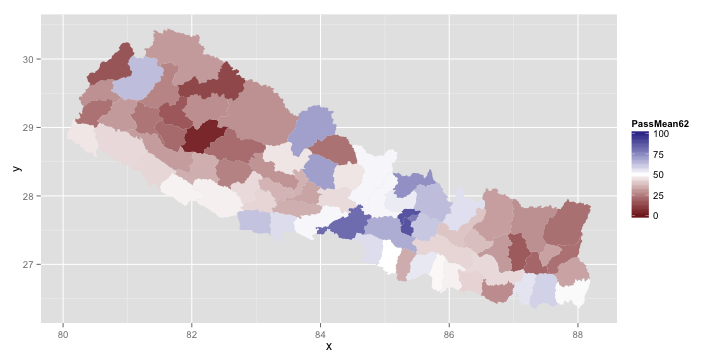Beautiful. Looks like a lot of change… can we see exactly what changed between the two years? (Notice that midpoint and range have to be changed here.)

districtavg <- merge(districtpassavg62, districtpassavg63, by = "District")
districtavg$delta <- districtavg$PassMean63 - districtavg$PassMean62 ggplot() + geom_map(data = districtavg, aes(map_id = District, fill = delta), map = np_dist) + expand_limits(x = np_dist$long, y = np_dist$lat) + scale_fill_gradient2(low = muted("red"), mid = "white", midpoint = 0, high = muted("blue"), limits = c(-20, 60))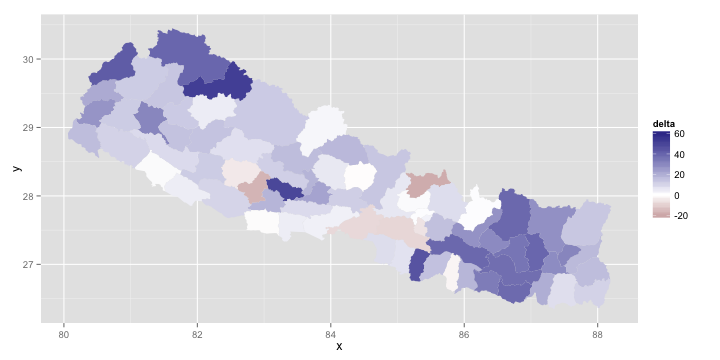Wow, looks like there was significant change in the eastern part of the country, as well as the far west, and at least one district west of center! ## Final bonus: district names on the map! But but… which districts are changing? Lets put some district names on the map. Again, here is where we appreciate R's generic data and plotting libraries… we can do this by basically making a dataset that creates centroids per district, and map a layer of text onto this map using that district-centers dataset. distcenters <- ddply(np_dist, .(id), summarize, clat = mean(lat), clong = mean(long)) ggplot() + geom_map(data = districtavg, aes(map_id = District, fill = delta), map = np_dist) + expand_limits(x = np_dist$long, y = np_dist\$lat) + scale_fill_gradient2(limits = c(-20,
60), low = muted("red"), mid = "white", midpoint = 0, high = muted("blue")) +
geom_text(data = distcenters, aes(x = clong, y = clat, label = id, size = 0.2))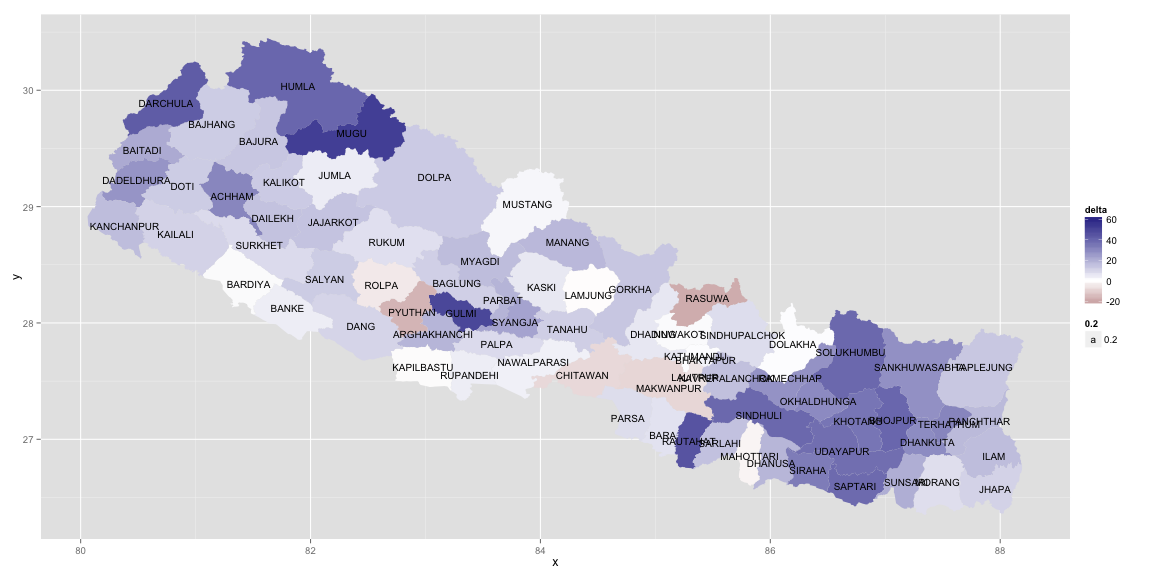Not perfect, but not bad, for an 20 lines of code (if I don't count purely demonstrational code) or so of interactive prototyping!